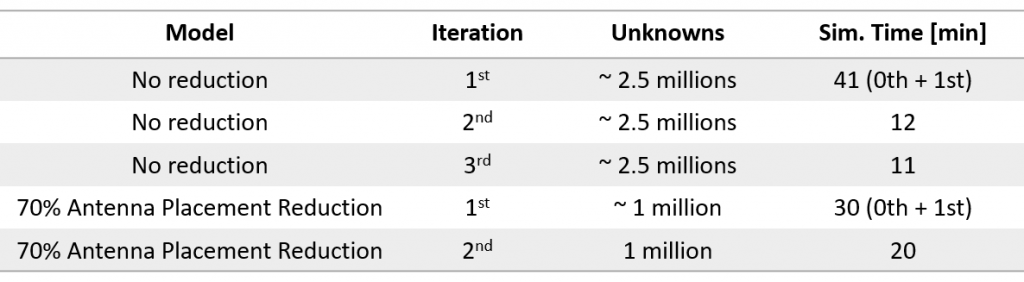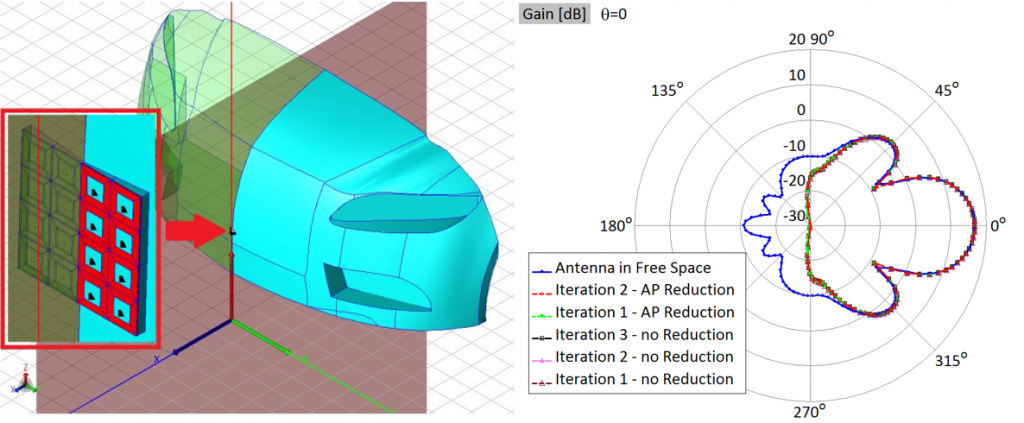Domain Decomposition Solver (DDS) can be used to solve very large problems typically encountered when dealing with complex antenna placement scenarios or RCS calculation, which can both easily slip beyond the reach of the direct MoM Solver.

Method description

The basic idea behind DDS is to divide the original problem in a number of groups. A group is composed of a number of neighboring plates and wires. Basis functions, which correspond to plates and wires from the same group, are grouped into a macro-basis function (MBFs).
All MBFs are multiplied by corresponding weighting coefficients, then replaced into full MoM matrix and residua of all equations are calculated. Weights of MBFs are determined by minimizing residuum of full MoM matrix. The residuum of the final solution in each iteration is used as the excitation in the next iteration. MBFs excited by low values of residua can be eliminated in iterative procedure, which significantly reduce simulation time with negligible influence to simulation accuracy.
The entire iterative procedure finishes when the total residuum becomes smaller than the predefined threshold.
In the 0th iteration, subprojects are simulated independently and the coupling between them is not taken into account. Groups which are simulated in the 0th iteration are:

• the groups which contain excitations – for the case of antenna placement problems,
• the groups directly illuminated by exciting plane waves – for the case of RCS problems.

The method converges rapidly and the solution with acceptable accuracy is typically found after 1 to 3 iterations.

Problem with 5 million unknowns (more than 1,000 wavelengths in size) can be solved in one day on multi-core desktop machine.

A good example demonstrating the power and efficiency of DDS solver can be the calculation of a radiation pattern of an anti-collision radar antenna (4×4 patch array), operating at the frequency of 77 GHz, placed on a car bumper. The number of unknowns and simulation times are given below.For more info on Domain Decomposition Solver and related application areas, please check the following application notes or publications: